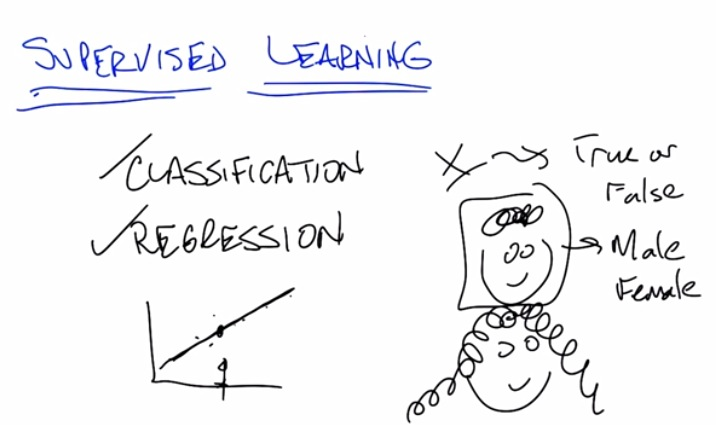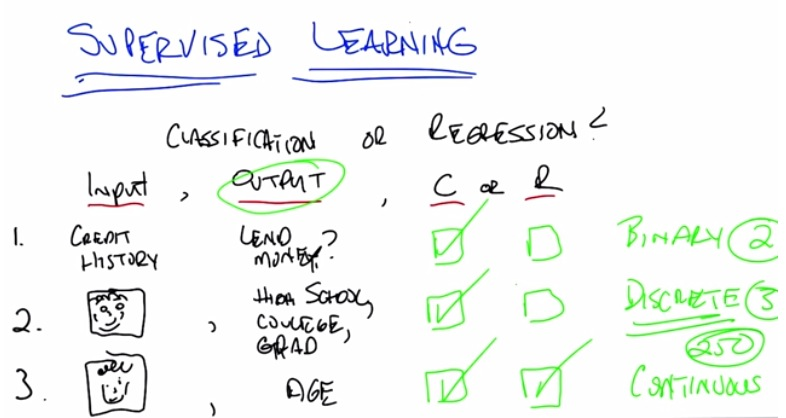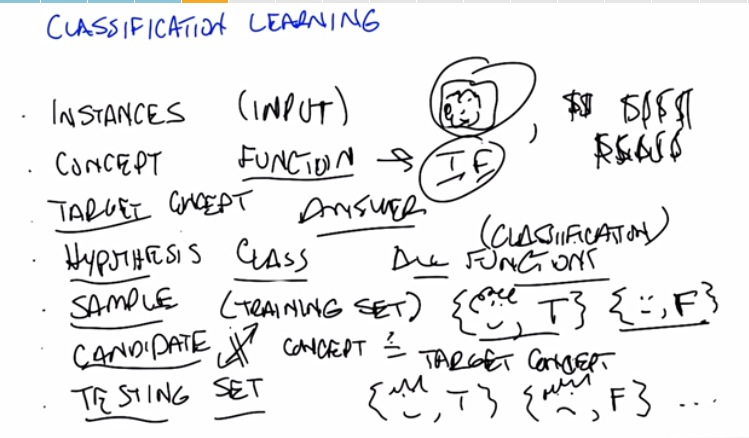• Classification is map the input to some set of discrete label (small value)
• Regression is map the input to real number/continous (often infinite number of value)
• Both can differentiate by how we look at the output• Third number is depend how we define the output. Is it large number of descrete value, or really just contiunuous. But because instructor said it is can be number of anything (fraction included) it should be regression.• An Instances, is an input a set of object that we have
• Concept is a function, a mapping that takes an input and mapped it to the output
• Target concept is an output, an output that is we're trying to represent
• Hypothesis is a class, a classification function that we're trying to be mapped
• The sample is training set, set of input and output, that build our function(concept)
• Candidate, is a rules that we have induct based on our training set. this is the candidate function that we use to mapped  output from input from our training set.
• The testing set, is part of our data, that doesn't used at all with training set. Here we want to test whether our candidate function have figured at all what are the generalized function or not. Based on this we want to know how correct our candicate function by testing it on the test set.